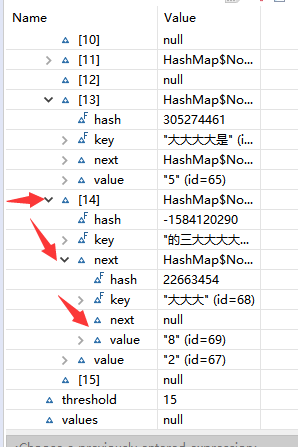﻿ HashMap原理及put方法与get方法的调用过程_java_脚本之家
java# HashMap原理及put方法与get方法的调用过程

## HashMap的原理

HashMap的数据结构为数组+链表，以key，value的形式存值，通过调用put与get方法来存值与取值。## HashMap底层原理(当你put,get时内部会发生什么呢?)cap```/**
* Associates the specified value with the specified key in this map.
* If the map previously contained a mapping for the key, the old
* value is replaced.
*
* @param key key with which the specified value is to be associated
* @param value value to be associated with the specified key
* @return the previous value associated with <tt>key</tt>, or
*         <tt>null</tt> if there was no mapping for <tt>key</tt>.
*         (A <tt>null</tt> return can also indicate that the map
*         previously associated <tt>null</tt> with <tt>key</tt>.)
*/
public V put(K key, V value) {
return putVal(hash(key), key, value, false, true);
}```

HashMap 采用一种所谓的“Hash 算法”来决定每个元素的存储位置。当程序执行 map.put(String,Obect)方法 时，系统将调用String的 hashCode() 方法得到其 hashCode 值——每个 Java 对象都有 hashCode() 方法，都可通过该方法获得它的 hashCode 值。得到这个对象的 hashCode 值之后，系统会根据该 hashCode 值来决定该元素的存储位置。小伙伴可以试试调用hashCode()方法看看经过此算法会得出怎样的结果.

(下面我们将每个下标统称为Entry(桶),也就是一个 key-value 对)

```/**
* Implements Map.put and related methods
*
* @param hash hash for key
* @param key the key
* @param value the value to put
* @param onlyIfAbsent if true, don't change existing value
* @param evict if false, the table is in creation mode.
* @return previous value, or null if none
*/
final V putVal(int hash, K key, V value, boolean onlyIfAbsent,
boolean evict) {
Node<K,V>[] tab; Node<K,V> p; int n, i;
if ((tab = table) == null || (n = tab.length) == 0)
n = (tab = resize()).length;
if ((p = tab[i = (n - 1) & hash]) == null)
tab[i] = newNode(hash, key, value, null);
else {
Node<K,V> e; K k;
if (p.hash == hash &&
((k = p.key) == key || (key != null && key.equals(k))))
e = p;
else if (p instanceof TreeNode)
e = ((TreeNode<K,V>)p).putTreeVal(this, tab, hash, key, value);
else {
for (int binCount = 0; ; ++binCount) {
if ((e = p.next) == null) {
p.next = newNode(hash, key, value, null);
if (binCount >= TREEIFY_THRESHOLD - 1) // -1 for 1st
treeifyBin(tab, hash);
break;
}
if (e.hash == hash &&
((k = e.key) == key || (key != null && key.equals(k))))
break;
p = e;
}
}
if (e != null) { // existing mapping for key
V oldValue = e.value;
if (!onlyIfAbsent || oldValue == null)
e.value = value;
afterNodeAccess(e);
return oldValue;
}
}
++modCount;
if (++size > threshold)
resize();
afterNodeInsertion(evict);
return null;
}```

```/**
* Replaces all linked nodes in bin at index for given hash unless
* table is too small, in which case resizes instead.
*/
final void treeifyBin(Node<K,V>[] tab, int hash) {
int n, index; Node<K,V> e;
// 如果数组等于null 或 数组长度小于 64
if (tab == null || (n = tab.length) < MIN_TREEIFY_CAPACITY)
// 重新散列，使得链表变短
resize();
// 如果hash冲突，且数组长度大于 64，则只能使用红黑树结构
else if ((e = tab[index = (n - 1) & hash]) != null) {
TreeNode<K,V> hd = null, tl = null;
// 返回新的红黑树
do {
TreeNode<K,V> p = replacementTreeNode(e, null);
if (tl == null)
hd = p;
else {
p.prev = tl;
tl.next = p;
}
tl = p;
} while ((e = e.next) != null);
if ((tab[index] = hd) != null)
hd.treeify(tab);
}
}```

1、容量太小。容量小，碰撞的概率就高了。狼多肉少，就会发生争强。

2、hash算法不够好。算法不合理，就可能都分到同一个或几个桶中。分配不均，也会发生争抢。

```public class HashTest {
public static void main(String[] args) {
// 对"负载因子的大小对程序的影响规律"进行测试
// threshold=capacity * loadFactor ---- 阈值 = 容量 x 负载因子
// 源代码扩容后容量是扩容前的二倍
int n1 = 10;   // 对照组
int n2 = 1000000; // put/get多少组
long t0 = 0;      //总耗时
float lf = 0.9f;  //负载因子
int capacity = 100; //初始容量
HashMap map = null;

//对照组循环
for (int j = 1; j <= n1; j++) {
map = new HashMap(capacity, lf);
List<String> list = new ArrayList<String>();
// 利用循环进行put
for (int i = 0; i < n2; i++) {
String temp = HashTest.randomString();
map.put(temp, i);
}
long time = 0; // 总耗费时间
// 利用循环get
for (int i = 0; i < n2; i++) {
String temp = list.get(i);
long t1 = System.currentTimeMillis();
map.get(temp);
long t2 = System.currentTimeMillis();
long t3 = t2 - t1;// 花费时间
time += t3;
}
System.out.println("组"+j+"花费时间(ms)=" + time);
t0 += time;
map = null;
}
System.out.println("get出 "+n2+" 对键值对中，"+n1+"组数据得出:");
System.out.println("---------------------------------");
System.out.println("每get"+n2+"对键值对 平均花费时间(毫秒)："+(t0/n1));
}
/**
* 产生随机字符串方法
* @return
*/
public static String randomString() {
// 最终产生的字符串
StringBuffer sb = new StringBuffer();
// 字符串样本
String str = "回到家卡萨恒大帝景阿萨德节快乐就看见了困窘企业无辜的鄙视你别这么想按一个预告的哈上东国际按时大大伽伽汇顶科技啊啥看的撒打算大的欧亚报出去qwertyuiopasdfghjklzxvcbnm,.;p[']/\1234567890zxcvbnmaksjhfgdlpoiuytrewq阿斯加德克拉斯近段时间的书上方法更符合辅导费的冠福股份极乐空间流口水";
// System.out.println("样本字符串长度:"+str.length());
// 产生一个1到30的数字
int num = (int) (Math.random() * 30 + 1);
// System.out.println("num="+num);
// 用for循环从样本字符串中提取出字符进行组合
for (int i = 0; i < num; i++) {
int num1 = (int) (Math.random() * str.length()); // 产生一个0到字符串样本的数字
// 根据索引值获取对应的字符
char charAt = str.charAt(num1);
sb.append(charAt);
}
// System.out.println("产生一个长度为"+num+"的字符串");
return sb.toString();
}```

Jdk的源代码，每一行都很有意思，都值得花时间去钻研、推敲。